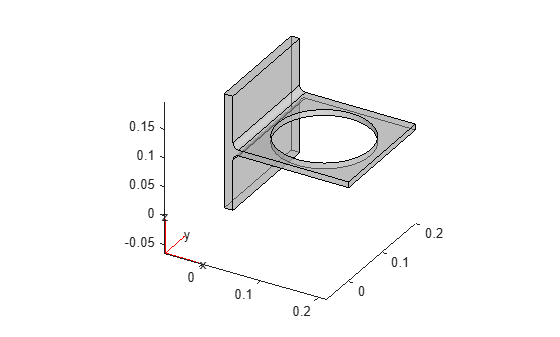# structuralProperties

Assign structural properties of material for structural model

## Syntax

``structuralProperties(structuralmodel,'YoungsModulus',YMval,'PoissonsRatio',PRval)``
``structuralProperties(___,'MassDensity',MDval)``
``structuralProperties(___,'CTE',CTEval)``
``structuralProperties(___,'HystereticDamping',g)``
``structuralProperties(___,RegionType,RegionID)``
``mtl = structuralProperties(___)``

## Description

````structuralProperties(structuralmodel,'YoungsModulus',YMval,'PoissonsRatio',PRval)` assigns Young's modulus and Poisson's ratio for the entire geometry. Use this syntax if your model is static and does not account for gravitational and thermal effects. TipA structural model supports only homogeneous isotropic materials. Therefore, all material properties must be numeric scalars. ```

example

````structuralProperties(___,'MassDensity',MDval)` assigns the mass density of the material for the entire geometry, and can include any of the arguments used in the previous syntax. Specify the mass density of the material if your model is transient or modal, or if it accounts for gravitational effects.```

example

````structuralProperties(___,'CTE',CTEval)` assigns the coefficient of thermal expansion for a thermal stress analysis. Use this syntax if your model is static and accounts for thermal effects.```

example

````structuralProperties(___,'HystereticDamping',g)` assigns the hysteretic damping parameter that models damping forces counteracting velocity but independent of frequency. Use this syntax for direct and modal frequency response analyses.```

example

````structuralProperties(___,RegionType,RegionID)` assigns material properties for the specified geometry region.```
````mtl = structuralProperties(___)` returns the material properties object.```

## Examples

collapse all

Create a structural model.

`structuralModel = createpde('structural','static-solid');`

Import and plot the geometry.

```importGeometry(structuralModel,'BracketWithHole.stl'); pdegplot(structuralModel,'FaceAlpha',0.5)```Specify Young's modulus, Poisson's ratio, and the mass density.

```structuralProperties(structuralModel,'YoungsModulus',200e9, ... 'PoissonsRatio',0.3, ... 'MassDensity',7800)```
```ans = StructuralMaterialAssignment with properties: RegionType: 'Cell' RegionID: 1 YoungsModulus: 2.0000e+11 PoissonsRatio: 0.3000 MassDensity: 7800 CTE: [] HystereticDamping: [] ```

Create a structural model for modal analysis.

`structuralModel = createpde('structural','modal-solid');`

Create and plot the geometry.

```gm = multicuboid(0.5,0.1,0.1); structuralModel.Geometry = gm; pdegplot(structuralModel,'FaceAlpha',0.5)```Specify Young's modulus, Poisson's ratio, and the mass density.

```structuralProperties(structuralModel,'YoungsModulus',210E3, ... 'PoissonsRatio',0.3, ... 'MassDensity',2.7E-6)```
```ans = StructuralMaterialAssignment with properties: RegionType: 'Cell' RegionID: 1 YoungsModulus: 210000 PoissonsRatio: 0.3000 MassDensity: 2.7000e-06 CTE: [] HystereticDamping: [] ```

Specify the coefficients of thermal expansion for a bimetallic cantilever beam. The bottom layer is steel. The top layer is copper.

Create a static structural model.

`structuralmodel = createpde('structural','static-solid');`

Create and plot the geometry.

```gm = multicuboid(0.5,0.04,[0.03,0.03],'Zoffset',[0,0.03]); structuralmodel.Geometry = gm; pdegplot(structuralmodel,'CellLabels','on')```Specify Young's modulus, Poisson's ratio, and the coefficient of thermal expansion for the bottom cell C1.

```structuralProperties(structuralmodel,'Cell',1', ... 'YoungsModulus',210e9, ... 'PoissonsRatio',0.28, ... 'CTE',1.3e-5)```
```ans = StructuralMaterialAssignment with properties: RegionType: 'Cell' RegionID: 1 YoungsModulus: 2.1000e+11 PoissonsRatio: 0.2800 MassDensity: [] CTE: 1.3000e-05 HystereticDamping: [] ```

Specify Young's modulus, Poisson's ratio, and the coefficient of thermal expansion for the top cell C2.

```structuralProperties(structuralmodel,'Cell',2', ... 'YoungsModulus',110e9, ... 'PoissonsRatio',0.37, ... 'CTE',2.4e-5)```
```ans = StructuralMaterialAssignment with properties: RegionType: 'Cell' RegionID: 2 YoungsModulus: 1.1000e+11 PoissonsRatio: 0.3700 MassDensity: [] CTE: 2.4000e-05 HystereticDamping: [] ```

Create a frequency response analysis model for a 3-D problem.

`structuralmodel = createpde('structural','frequency-solid');`

Create nested cylinders to model a bimetallic cable.

`gm = multicylinder([0.01,0.015],0.05);`

Assign the geometry to the structural model and plot the geometry.

```structuralmodel.Geometry = gm; pdegplot(structuralmodel,'CellLabels','on','FaceAlpha',0.4)```Specify Young's modulus, Poisson's ratio, mass density, and hysteretic damping for both cylinders.

```structuralProperties(structuralmodel,'YoungsModulus',110E9, ... 'PoissonsRatio',0.28, ... 'MassDensity',7800, ... 'HystereticDamping',0.2, ... 'Cell',1)```
```ans = StructuralMaterialAssignment with properties: RegionType: 'Cell' RegionID: 1 YoungsModulus: 1.1000e+11 PoissonsRatio: 0.2800 MassDensity: 7800 CTE: [] HystereticDamping: 0.2000 ```
```structuralProperties(structuralmodel,'YoungsModulus',210E9, ... 'PoissonsRatio',0.3, ... 'MassDensity',7800, ... 'HystereticDamping',0.1, ... 'Cell',2)```
```ans = StructuralMaterialAssignment with properties: RegionType: 'Cell' RegionID: 2 YoungsModulus: 2.1000e+11 PoissonsRatio: 0.3000 MassDensity: 7800 CTE: [] HystereticDamping: 0.1000 ```

Create a structural model.

`structuralModel = createpde('structural','static-solid');`

Create nested cylinders to model a bimetallic cable.

`gm = multicylinder([0.01,0.015],0.05);`

Assign the geometry to the structural model and plot the geometry.

```structuralModel.Geometry = gm; pdegplot(structuralModel,'CellLabels','on','FaceAlpha',0.4)```Specify Young's modulus and Poisson's ratio for each metal.

```structuralProperties(structuralModel,'Cell',1,'YoungsModulus',110E9, ... 'PoissonsRatio',0.28)```
```ans = StructuralMaterialAssignment with properties: RegionType: 'Cell' RegionID: 1 YoungsModulus: 1.1000e+11 PoissonsRatio: 0.2800 MassDensity: [] CTE: [] HystereticDamping: [] ```
```structuralProperties(structuralModel,'Cell',2,'YoungsModulus',210E9, ... 'PoissonsRatio',0.3)```
```ans = StructuralMaterialAssignment with properties: RegionType: 'Cell' RegionID: 2 YoungsModulus: 2.1000e+11 PoissonsRatio: 0.3000 MassDensity: [] CTE: [] HystereticDamping: [] ```

## Input Arguments

collapse all

Structural model, specified as a `StructuralModel` object. The model contains the geometry, mesh, structural properties of the material, body loads, boundary loads, and boundary conditions.

Example: ```structuralmodel = createpde('structural','transient-solid')```

Young's modulus of the material, specified as a positive number.

Example: `structuralProperties(structuralmodel,'YoungsModulus',210e3,'PoissonsRatio',0.3)`

Data Types: `double`

Poisson's ratio of the material, specified as a number greater than 0 and less than 0.5.

Example: `structuralProperties(structuralmodel,'YoungsModulus',210e3,'PoissonsRatio',0.3)`

Data Types: `double`

Mass density of the material, specified as a positive number. This argument is required for transient and modal models. `MDval` is also required when modeling gravitational effects.

Example: `structuralProperties(structuralmodel,'YoungsModulus',210e3,'PoissonsRatio',0.3,'MassDensity',11.7e-6)`

Data Types: `double`

Coefficient of thermal expansion, specified as a real number. This argument is required for thermal stress analysis. Thermal stress analysis requires the structural model to be static.

Example: `structuralProperties(structuralmodel,'YoungsModulus',210e3,'PoissonsRatio',0.3,'MassDensity',2.7e-6,'CTE',11.7e-6)`

Data Types: `double`

Hysteretic damping, specified as a nonnegative number. This type of damping is also called structural damping.

Example: `structuralProperties(structuralmodel,'YoungsModulus',210E9,'PoissonsRatio',0.3,'MassDensity',7800,'HystereticDamping',0.1)`

Data Types: `double`

Geometric region type, specified as `'Face'` for a 2-D model or `'Cell'` for a 3-D model.

Example: `structuralProperties(structuralmodel,'Cell',1,'YoungsModulus',110E9,'PoissonsRatio',0.3)`

Data Types: `char` | `string`

Geometric region ID, specified as a vector of positive integers. Find the region IDs using `pdegplot` with the `'FaceLabels'` (3-D) or `'EdgeLabels'` (2-D) value set to `'on'`.

Example: `structuralProperties(structuralmodel,'Cell',1:3,'YoungsModulus',110E9,'PoissonsRatio',0.3)`

Data Types: `double`

## Output Arguments

collapse all

Handle to material properties, returned as a `StructuralMaterialAssignment` object. See StructuralMaterialAssignment Properties.

`mtl` associates the material properties with the geometric region.

## Version History

Introduced in R2017b